## Isothermal Reaction

Original source is K. D. Williamowski, O. E. Rössler (1979), quoted in: C. B. Smith, B. Kuszta, G. Lyberatos, J. E. Bailey: Period doubling and complex dynamics in an isothermal chemical reaction system. Chem. Eng. Sci. 38 (1983) 425-430.

Four parameter values of period doubling are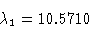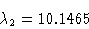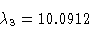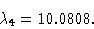The expected accumulation point is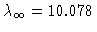.

Figure 1
Bifurcation diagram, y1(0) versus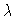.

Figure 2
Isothermal reaction, periodic orbit,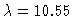, projection to (y1,y2) plane.

Figure 3
Isothermal reaction, periodic orbit,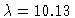, projection to (y1,y2) plane.

Figure 4
Isothermal reaction, chaotic orbit,, projection to (y1,y2) plane.

This Example
Figure 1
Figure 2
Figure 3
Figure 4